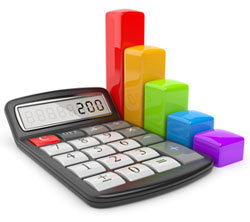JavaScript must be turned on in order for this site to display properly.

# Calculating Finance Charges

## How finance charges and interest are calculated on a DCU loan

### DCU Visa® Credit Card Finance Charges

• Interest (Finance Charge) is a fee charged on every Visa account that is not paid in full by the payment due date or on every Visa account that has a cash advance.
• The Finance Charge formula is:

Average Daily Balance  x  Annual Percentage Rate (APR)  x  Number of Days in Billing Cycle   ÷  365

• To determine your Average Daily Balance:

Add up the end-of-the-day balances for every day of the billing cycle. You can find the dates of the billing cycle on your monthly Visa Statement. Divide the total of the end-of-the-day balances by the number of days in the billing cycle. This is your Average Daily Balance.

#### Sample Calculation

Assume Average Daily Balance of 1,322.58 with a 9.9% Annual Percentage Rate in a 31-day billing cycle.

Total Finance Fee Due for the Billing Cycle  =  \$1,322.58  x .099%  x  31  ÷  365  =  \$11.12

### Closed End (non-revolving) Consumer and Mortgage Loans

Closed end loans are loans for a specific dollar amount, that you agree to pay back within a certain period of time (usually years). Usually you're agreeing to pay the money back according to an 'amortization' schedule.

Amortization is simply the reducing of a debt through periodic payments. Basically, you're dividing the total number of payments into the amount you've borrowed plus interest. Each month a portion of the loan payment you make will go towards reducing the principal amount borrowed, and a portion will go towards reducing the total finance fee. You will see this breakdown by the headings on the loan activity portion of your DCU statement.

The monthly payments and finance charges will always be disclosed to you up front when you close on your loan but here's how to understand how these figures are calculated.

You'll need:

• the amount you are borrowing
• the term of the loan (in years)
• the number of payments due each year (always 12 at DCU)
• the Annual Percentage Rate (APR)

Using these numbers:

• Determine how many monthly payments you will make over the term of the loan (the term in years multiplied by the number of payments due each year – usually 12).
• Convert the APR to a decimal (APR% divided by 100.00).
• Then calculate the interest rate for each payment (because it is an annual rate, you will divide the rate by 12).

#### Formula 1

To calculate your monthly payment amount:

 Payment  = Interest rate due on each payment  x  amount borrowed 1  –  (1  +  Interest rate due on each payment)  –  Number of payments

##### Sample Calculation

Assume you have applied for an auto loan for \$15,000, for 5 years, at an annual rate of 7.20%

• Number of payments  =  5  x  12  =  60
• Interest rate as a decimal  =  7.20%  ÷  100  =  .072
• Interest due on each payment  =  .072  ÷  12  =  .006

Plug each into Formula 1 above:

 Payment  = .006  x  \$15,000 1  –  (1  +  .006)  –  60 =  \$298.44 monthly payment

#### Formula 2

To Calculate Total Finance Charges to be Paid:

Monthly Payment Amount  x  Number of Payments  –  Amount Borrowed  =  Total Amount of Finance Charges

##### Sample Calculation:

Plug each of the above into Formula 2 above:

• \$298.44  x  60  –  \$15,000.00  =  \$2,906.13

The figures for a mortgage will usually be quite a bit higher, but the basic formulas can still be used.

### Loan Calculators

We have an extensive collection of calculators on this site. You can use them to determine loan payments and create loan amortization sheets that break out the portion of each payment that goes to principal and interest over the life of a loan.